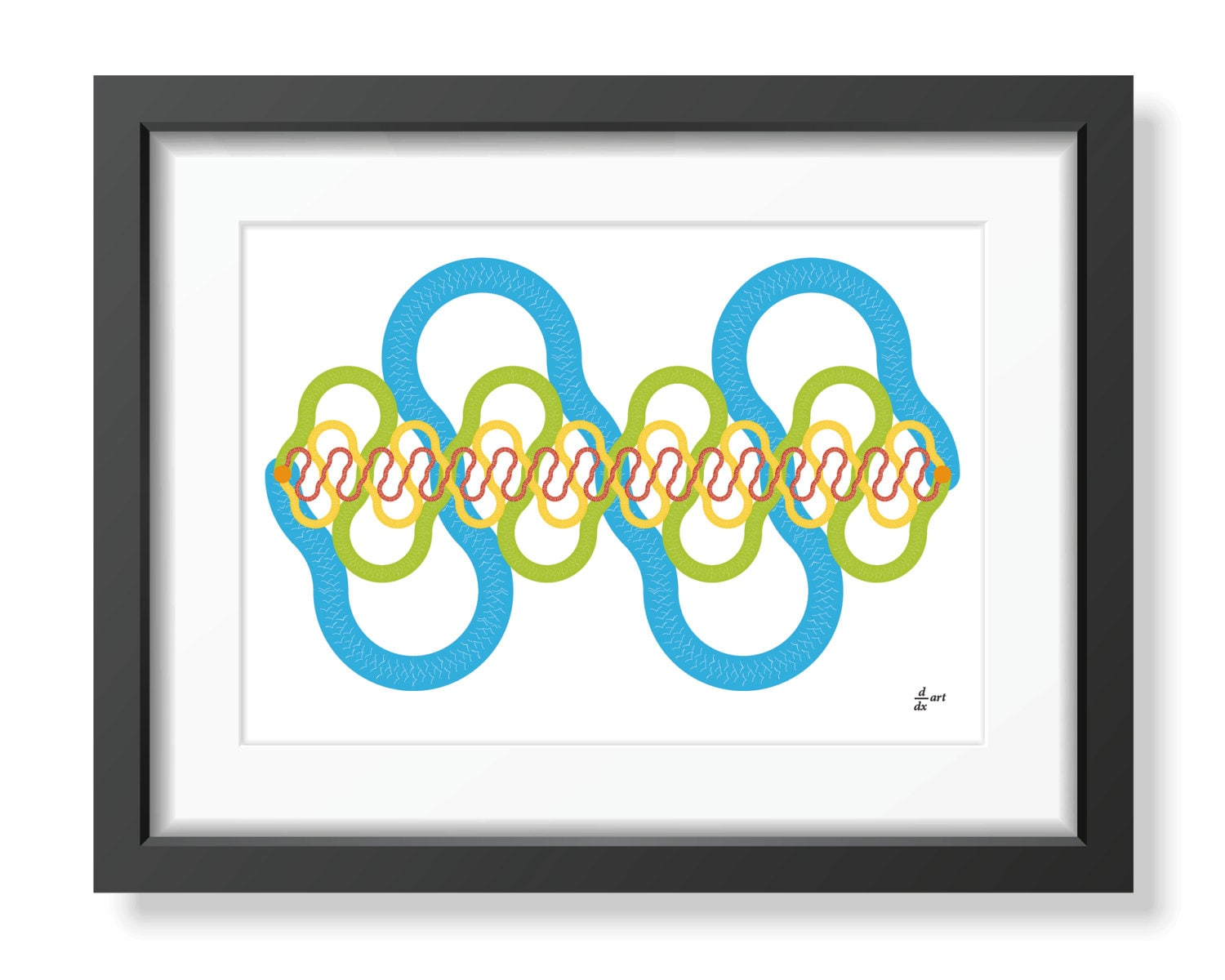First of all, apologies for the pun, but this simple and elegant design arose out of the strange fact that the ratio between the distance water flows along a river from source to the mouth and the straight line between those two points is often surprisingly close to the strange number called pi, and usually represented by the symbol π.

Most of us remember from school that π is the ratio between two apparently quite ordinary things – the diameter of a circle and its circumference. If you take a piece of string and measure across the widest part of a circle and then take that length and arrange it carefully around the circumference of the circle, that circumference will turn out to be in the region of three times the length of the string plus about another seventh.

Is the at best we can do? Well no; if you want to be precise about it, π has so far been calculated to something over 10 trillion decimal places, starting with 3.141592… and never ending.

π has many strange qualities apart from being impossible ever to pin down in terms of its decimal representation. It is also, for instance, an ‘irrational’ number, meaning it is not the ratio between two numbers. We often use 22/7 as an approximation, or even better, something clever like 333/106  but actually there is no combination of numbers that does the job.

But all that’s another story, the interesting thing in this case is just how often the ratio π, or something close to it, occurs in nature – every time something is circular – and in science, where all kinds of calculations which don’t appear to involve circles nevertheless require π somewhere in the mix.

This design celebrates the strange fact, mentioned above, that the average length of the rivers of the world, as measured by the distance the water flows, is close to 3.14 times the straight line distance.In our case we have very well-behaved semi-circular rivers but they make an interesting mathematical point, too. There are four different lines on the page, superimposed. The thickest one sweeps out towards the edge of the paper, the thinnest wiggles back and forth less energetically. But they are all made up of semi-circles based on the same straight line.

So have you guessed? Despite appearances they are all exactly the same length. In fact if you travel from the top to the bottom, you can change lines anywhere they cross without altering the overall distance.And because they are all half-circles, we know that the total distance is the length of the straight line times π and, in our case because they are half-circles, divided by two.

Recently I revisited the concept and produced something less elegant and minimalist but where the lines are (more or less!) PI times the straight line distance rather than the π/2 of the original.